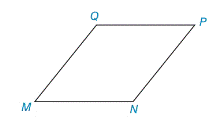Chapter 8.1, Problem 5EElementary Geometry For College St...

7th Edition
Alexander + 2 others
ISBN: 9781337614085

Solutions

Chapter
SectionElementary Geometry For College St...

7th Edition
Alexander + 2 others
ISBN: 9781337614085
Textbook Problem

In rhombus MNPQ, how does the length of the altitude from Q to P N ¯ compare to the length of the altitude from Q to M N ¯ ? Explain.Exercises 4–6

To determine

To Explain:

In rhombus MNPQ, the length of the altitude from Q to PN¯ and comparing it to the length of the altitude from Q to MN¯.

Explanation

Given,

In rhombus MNPQ,

The length of the altitude from Q to PN¯ is QR¯ and to the length of the altitude from Q to MN¯ is QS¯.

Find QR:

For the RPQ,

Here, PQ=x and RP=x2

By the Pythagorean theorem,

(RP)2+(QR)2=(PQ)2

(x2)2+(QR)2=(x)2

x24+(QR)2=(x)2

Add x24 on both sides,

x24+(QR)2x24=x2x24

(QR)2=4x2x24

(QR)2=3x24

QR=3x24

QR=32x

Fi

Still sussing out bartleby?

Check out a sample textbook solution.

See a sample solution

The Solution to Your Study Problems

Bartleby provides explanations to thousands of textbook problems written by our experts, many with advanced degrees!

Get Started

In Exercises 4756, solve the given equation for the indicated variable. 81=3x

Finite Mathematics and Applied Calculus (MindTap Course List)

Use the following matrices for Problems 1-28. 3. Which matrices have the same order as G?

Mathematical Applications for the Management, Life, and Social Sciences

Simplify: 918

Elementary Technical Mathematics

In Exercises 1-22, evaluate the given expression. C(7,5)

Finite Mathematics for the Managerial, Life, and Social Sciences

True or False: y′ + xey = ex+y is separable.

Study Guide for Stewart's Single Variable Calculus: Early Transcendentals, 8th

It does not exist.

Study Guide for Stewart's Multivariable Calculus, 8th